• 今天为大家带来数量关系：等差数列中项法求和的应用。【导读】中公事业单位为帮助各位考生顺利通过事业单位招聘考试！今天为大家带来数量关系：等差数列中项法求和的应用。下半年的事业单位考试正在来袭，在大家备考...
【导读】中公事业单位为帮助各位考生顺利通过事业单位招聘考试！今天为大家带来数量关系：等差数列中项法求和的应用。【导读】中公事业单位为帮助各位考生顺利通过事业单位招聘考试！今天为大家带来数量关系：等差数列中项法求和的应用。下半年的事业单位考试正在来袭，在大家备考过程中不难发现，计算问题属于一类高频考点，而计算问题中的等差数列又是这一类题里最常考的知识点，那么，今天和大家分享的是等差数列中中项法求和公式的应用。我们初中时就知道了等差数列的通项公式和求和公式，但是那时主要应用的求和公式是：前n项和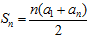，即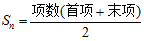，而在实际解题过程中我们发现，等差数列的中项法求和公式应用起来解题会更快。所以，对于等差数列的学习，一定要掌握中项法求和的方式。中项法求和分为两种情况，一是数列为奇数项时：Sn=中间一项×项数。【例1】主席台前排坐着5个人，最小的一个32岁，从第二个起，每个人都比前一个人年龄大3岁，则这个5个人的平均年龄为( )A.28 B.35 C.38 D.41【答案】C。【中公解析】方法一，依题意可知，5人年龄构成公差为3的等差数列，求5人的平均年龄，只需求5人的年龄和，再除以5即可，a1=32,根据通项公式易知a5=44，则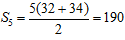，所以，5人平均年龄为190÷5=38。方法二，由中项法求和可知：五个人的年龄和S5=第三个人的年龄×5，所以第三个人的年龄即等于5人的平均年龄，第一人是32岁，则第二人为35岁，第三人为38岁，此题选C。中项法求和的另一种情况是数列为偶数项时：Sn=中间两项和×项数的一半。【例2】一张试卷共8道题，后面每一道题总比前一道多4分，如果试卷满分120分，那么第四道题分值是：A.17 B.16 C.13 D.11【答案】C。【中公解析】方法一，依题意，8道题的分值构成公差为4的等差数列，8项的和S8=120，根据通项公式和常规求和公式有：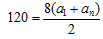……①;a8=a1+(8-1)×4……②;联立两式解得a1=1,所以，a4=1+(4-1)×4=13。方法二，由中项法求和可知：S8=120=(a4+a5)×4，则(a4+a5)=30，又因为a5比a4大4，所以a5=17，a4=13.此题选C.通过以上两道例题不难看出，在等差数列的计算问题中，如果能灵活运用中项法求和公式，那么解题过程也许会变得简单，更容易得到结果，所以，在事业单位备考过程中，一定要对中项法求和很熟悉，做到灵活运用。相关推荐
展开全文• 也可以用数学归纳证明，不过需要猜公式是什么。
也可以用数学归纳法证明，不过需要猜公式是什么。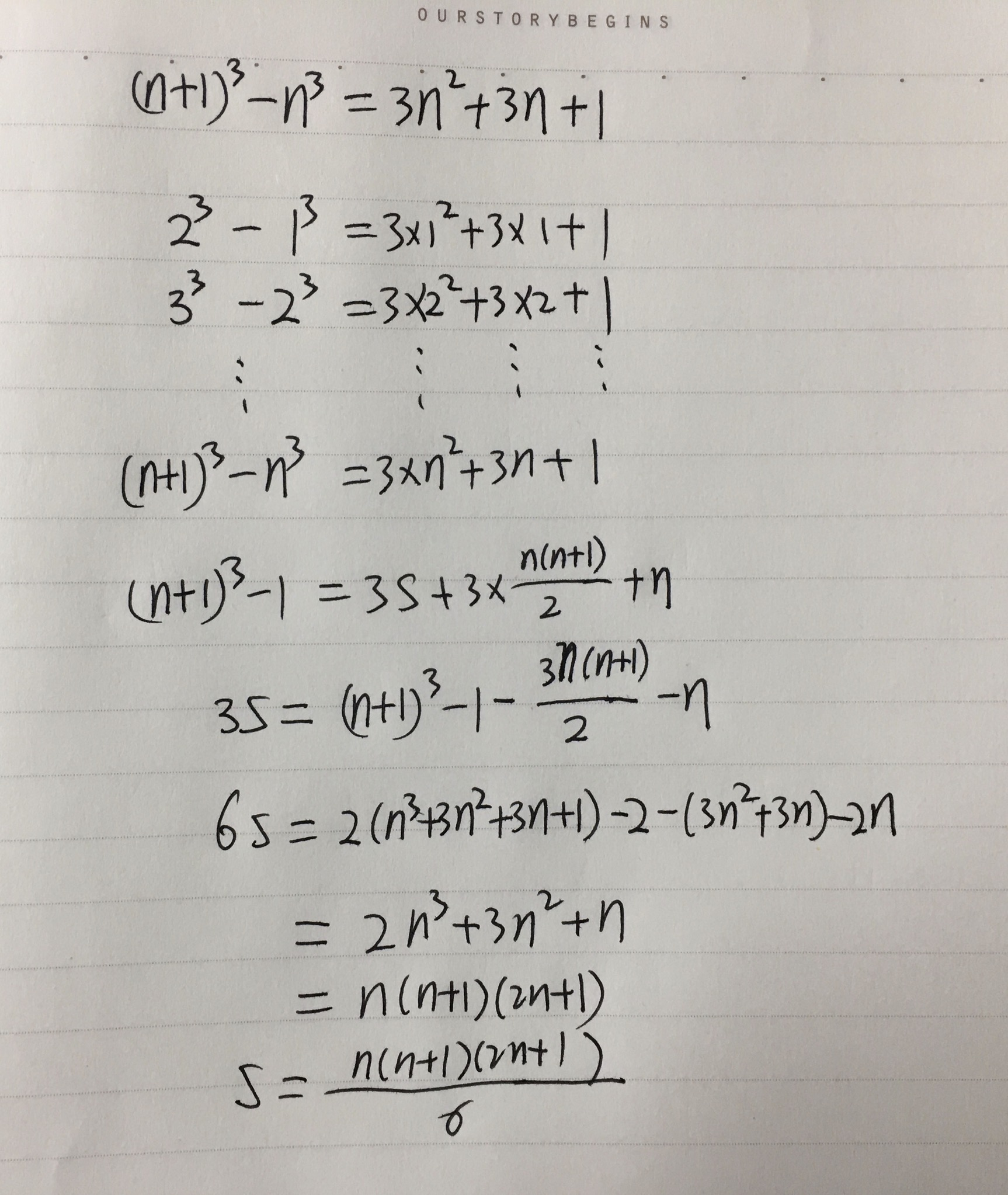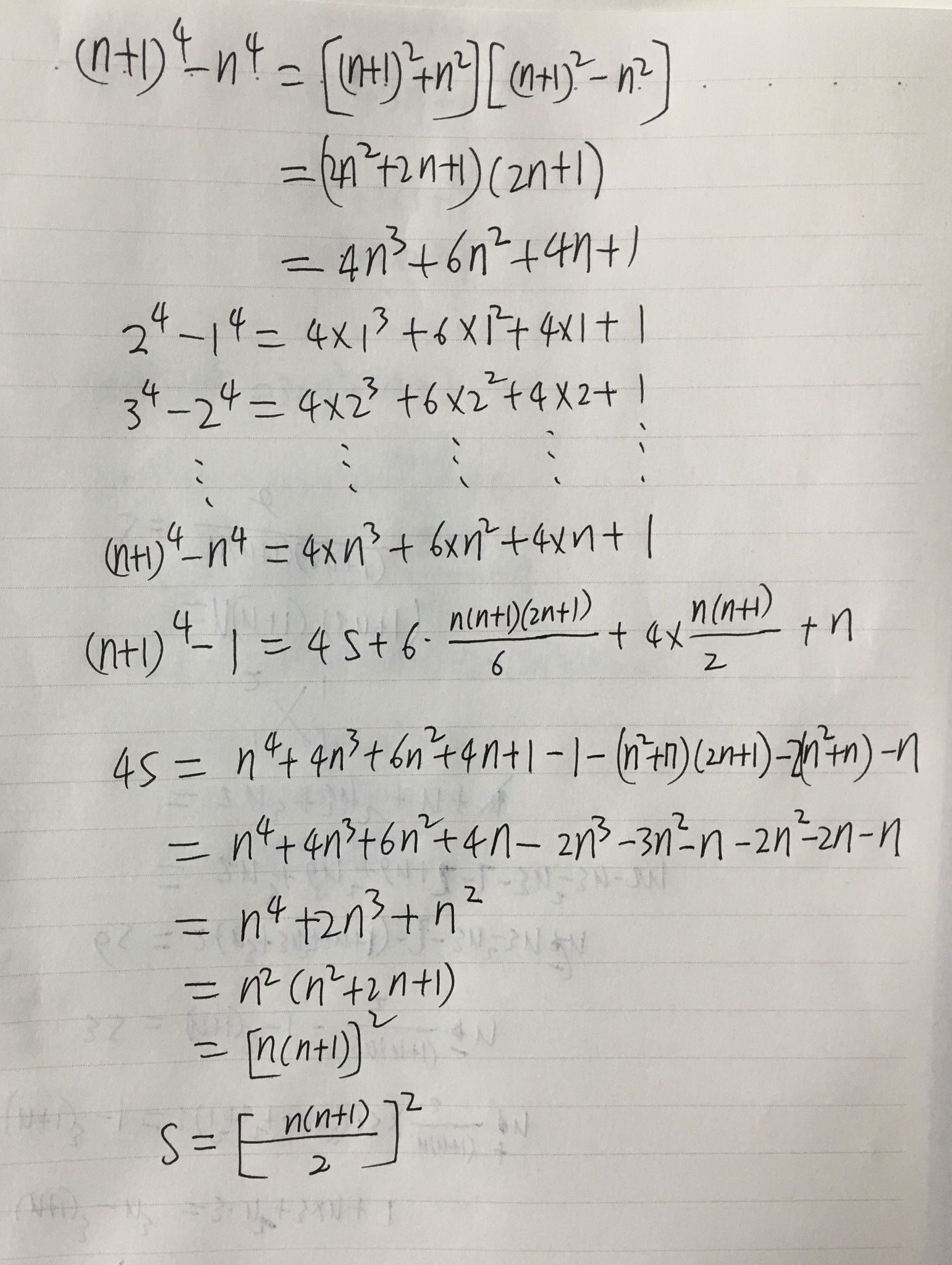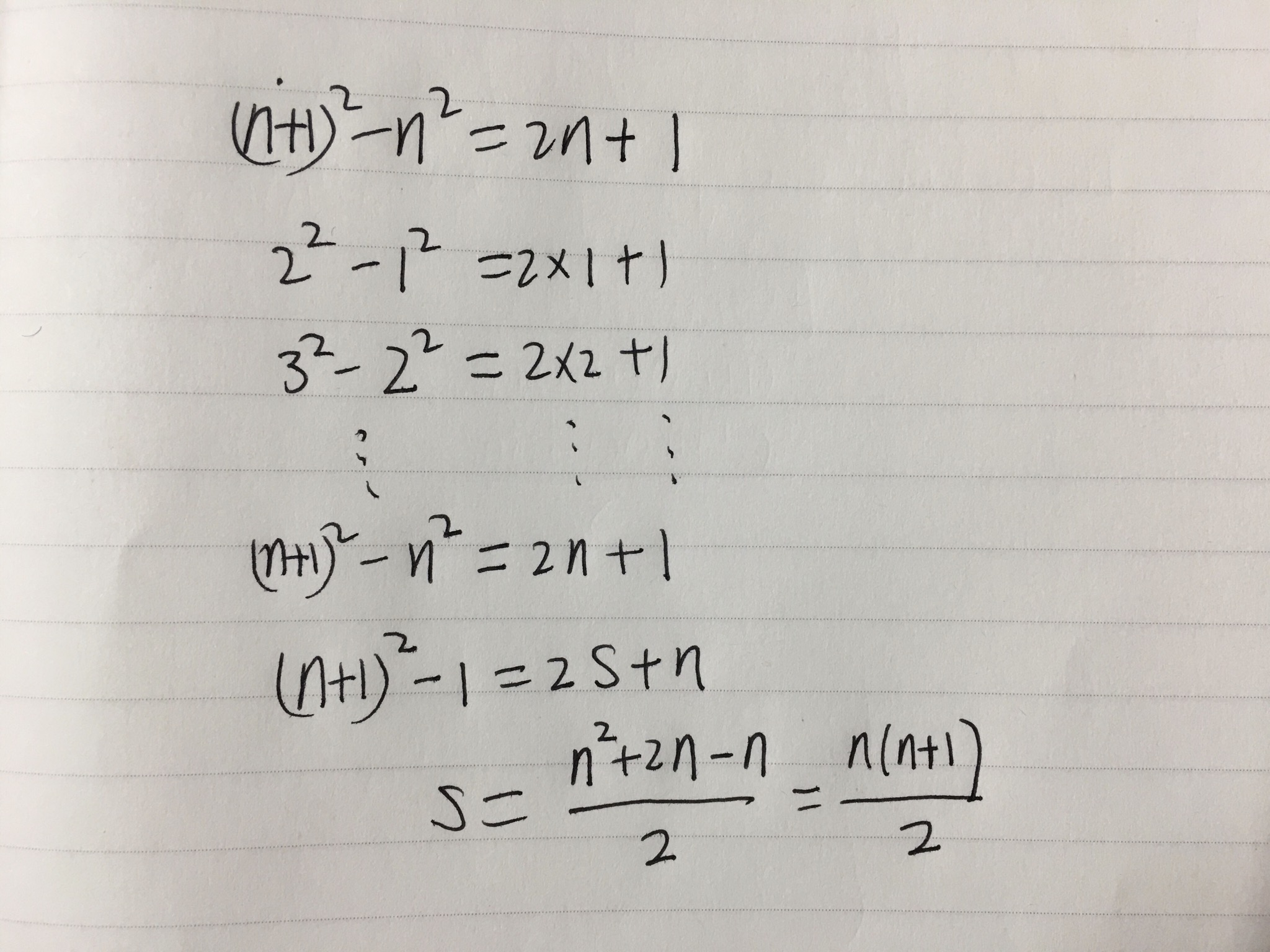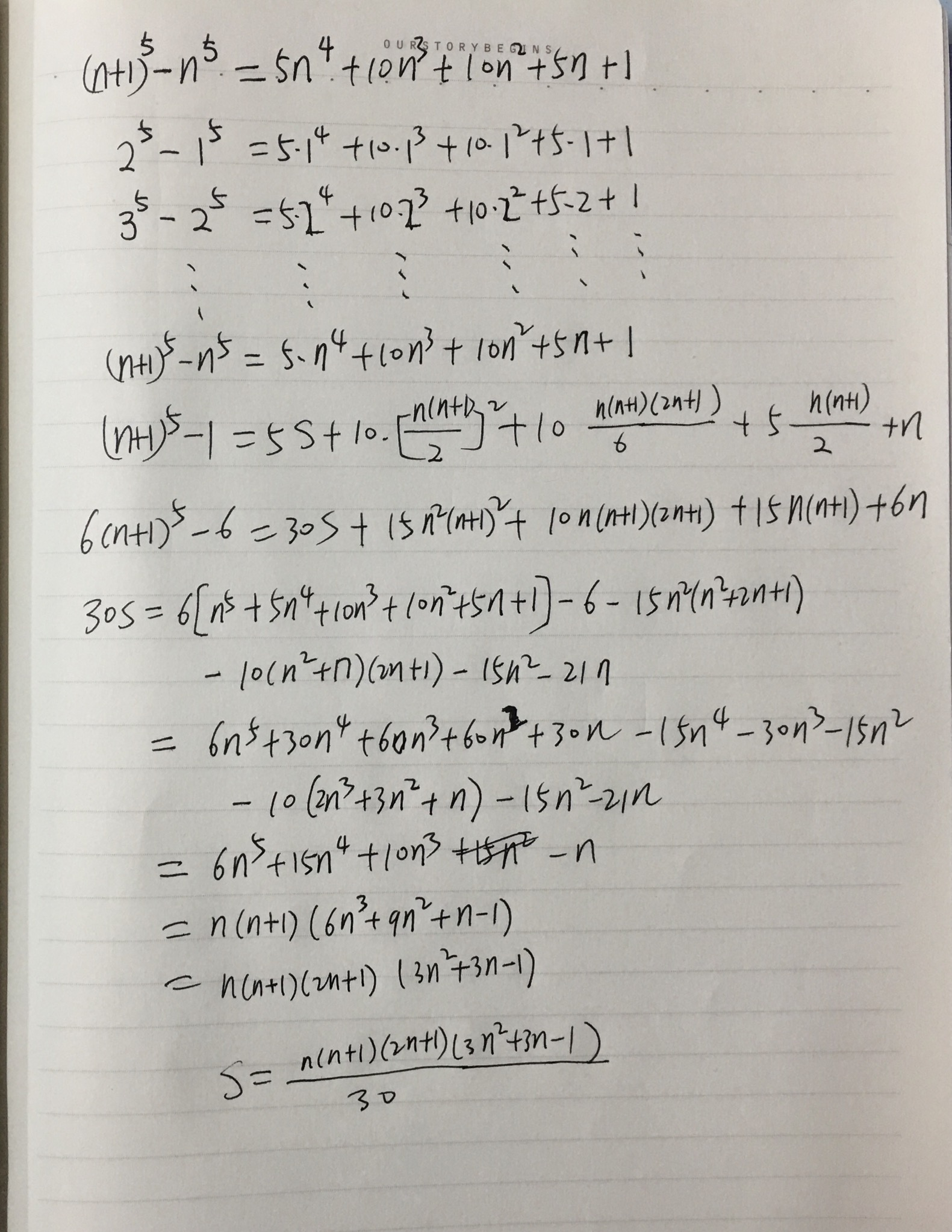展开全文• 2021届二轮复习 数学归纳数列的通项公式与数列求和 学案（全国通用）.doc
• 2021届二轮复习 数学归纳数列的通项公式与数列求和 课时作业（全国通用）.doc
• 等差数列求和公式1.公式法2.错位相减法3.求和公式4.分组有一类数列，既不是等差数列，也不是等比数列，若将这类数列适当拆开，可分为几个等差、等比或常见的数列，然后分别求和，再将其合并即可.5.裂相消法适用...
数学大师等差数列是常见数列的一种，可以用AP表示，如果一个数列从第二项起，每一项与它的前一项的差等于同一个常数，这个数列就叫做等差数列，而这个常数叫做等差数列的公差，公差常用字母d表示。等差数列求和公式1.公式法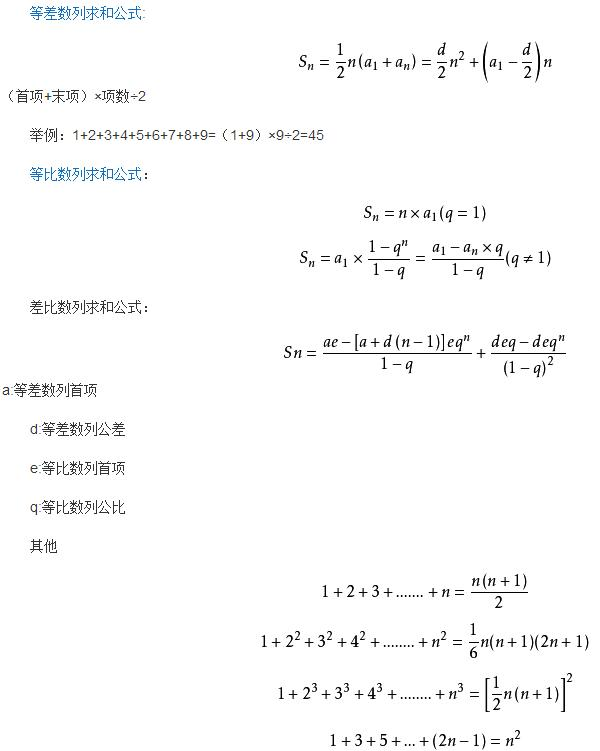2.错位相减法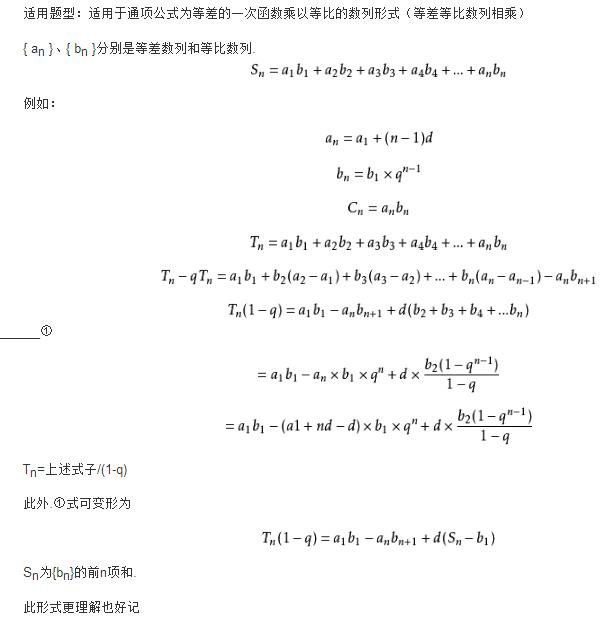3.求和公式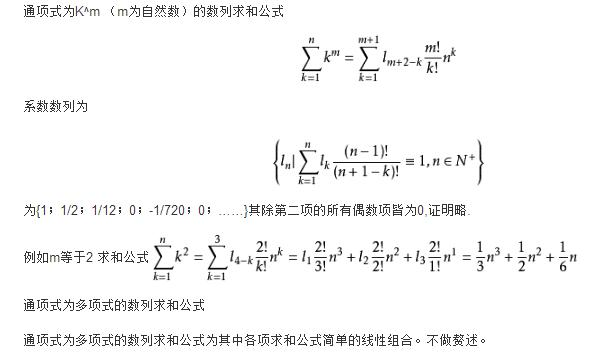4.分组法有一类数列，既不是等差数列，也不是等比数列，若将这类数列适当拆开，可分为几个等差、等比或常见的数列，然后分别求和，再将其合并即可.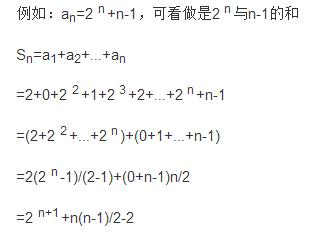5.裂项相消法适用于分式形式的通项公式，把一项拆成两个或多个的差的形式，即an=f(n+1)－f(n)，然后累加时抵消中间的许多项。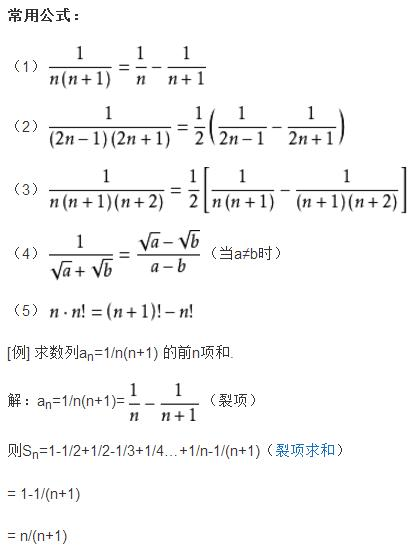【小结】此类变形的特点是将原数列每一项拆为两项之后，其中中间的大部分项都互相抵消了。只剩下有限的几项。注意：余下的项具有如下的特点1、余下的项前后的位置前后是对称的。2、余下的项前后的正负性是相反的。6.数学归纳法一般地，证明一个与正整数n有关的命题，有如下步骤：(1)证明当n取第一个值时命题成立；(2)假设当n=k(k≥n的第一个值，k为自然数)时命题成立，证明当n=k+1时命题也成立。【例】求证：1×2×3×4 + 2×3×4×5 + 3×4×5×6 + .…… + n(n+1)(n+2)(n+3) = [n(n+1)(n+2)(n+3)(n+4)]/5证明：当n=1时，有：1×2×3×4 = 24 = 2×3×4×5/5假设命题在n=k时成立，于是：1×2x3×4 + 2×3×4×5 + 3×4×5×6 + .…… + k(k+1)(k+2)(k+3) = [k(k+1)(k+2)(k+3)(k+4)]/5则当n=k+1时有：1×2×3×4 + 2×3×4×5 + 3×4×5×6 + …… + (k+1)(k+2)(k+3)(k+4)= 1×2×3×4 + 2×3×4*5 + 3×4×5×6 + …… + k(k+1)(k+2)(k+3) + (k+1)(k+2)(k+3)(k+4)= [k(k+1)(k+2)(k+3)(k+4)]/5 + (k+1)(k+2)(k+3)(k+4)= (k+1)(k+2)(k+3)(k+4)*(k/5 +1)= [(k+1)(k+2)(k+3)(k+4)(k+5)]/5即n=k+1时原等式仍然成立，归纳得证7.并项求和法(常采用先试探后求和的方法)【例】1－2+3－4+5－6+……+(2n-1)-2n方法一：(并项)求出奇数项和偶数项的和，再相减。方法二：(1－2)+(3－4)+(5－6)+……+[(2n-1)-2n]方法三：构造新的数列，可借用等差数列与等比数列的复合。an=n(-1)^(n+1)等差数列判定及其性质等差数列的判定(1)a(n+1)--a(n)=d (d为常数、n ∈N*)[或a(n)--a(n-1)=d，n ∈N*，n ≥2,d是常数]等价于{a(n)}成等差数列。(2)2a(n+1)=a(n)+a(n+2) [n∈N*] 等价于{a(n)}成等差数列。(3)a(n)=kn+b [k、b为常数,n∈N*] 等价于{a(n)}成等差数列。(4)S(n)=A(n)^2 +B(n) [A、B为常数，A不为0，n ∈N* ]等价于{a(n)}为等差数列。特殊性质在有穷等差数列中，与首末两项距离相等的两项和相等。并且等于首末两项之和；特别的，若项数为奇数，还等于中间项的2倍,即，a(1)+a(n)=a(2)+a(n-1)=a(3)+a(n-2)=···=2*a中【例】数列：1，3，5，7，9，11中a(1)+a(6)=12 ; a(2)+a(5)=12 ; a(3)+a(4)=12 ;即，在有穷等差数列中，与首末两项距离相等的两项和相等。并且等于首末两项之和。数列：1，3，5，7，9中a(1)+a(5)=10 ; a(2)+a(4)=10 ; a(3)=5=[a(1)+a(5)]/2=[a(2)+a(4)]/2=10/2=5 ; 即，若项数为奇数，和等于中间项的2倍，另见，等差中项。​数学大师
展开全文• 数列前n和(分组求和法) 精品文档 精品文档 收集于网络如有侵权请联系管理员删除 收集于网络如有侵权请联系管理员删除 精品文档 收集于网络如有侵权请联系管理员删除 1.求数列的前和 2.数列{an}的通项公式...
• ## 数列求和公式汇总

万次阅读 2017-08-06 18:51:07
常见公式 1+2+3+…+n=n(n+1)/2 ...{//等差数列求和公式 return (n+1)*n/2;//注意(n+1)/2*n这样不对 } q^1+q^2+q^3+…+q^n=q*(q^n-1)/(q-1) LL power(int a,int b){ LL ans=1,base=a; while(b&gt;0)...
常见公式

1+2+3+…+n=n(n+1)/2

LL getSum(LL n)
{//等差数列求和公式
return (n+1)*n/2;//注意(n+1)/2*n这样不对
}

q^1+q^2+q^3+…+q^n=q*(q^n-1)/(q-1)

LL power(int a,int b){
LL ans=1,base=a;
while(b>0){
if(b&1) //n%2==1
ans=ans*base;
base=base*base;
b>>=1;// b/=2
}
return ans;
}
LL getSum(LL q,LL n)
{//等比数列求和公式
return q*(power(q,n)-1)/(q-1);
}

1²+2²+3²…+n²=n(n+1)(2n+1)/6

推导过程

利用立方差公式
n^3-(n-1)^3=1*[n^2+(n-1)^2+n(n-1)]
=n^2+(n-1)^2+n^2-n
=2*n^2+(n-1)^2-n
2^3-1^3=2*2^2+1^2-2
3^3-2^3=2*3^2+2^2-3
4^3-3^3=2*4^2+3^2-4
.
n^3-(n-1)^3=2*n^2+(n-1)^2-n
各等式全相加
n^3-1^3=2*(2^2+3^2+…+n^2)+[1^2+2^2+…+(n-1)^2]-(2+3+4+…+n)
n^3-1=2*(1^2+2^2+3^2+…+n^2)-2+[1^2+2^2+…+(n-1)^2+n^2]-n^2-(2+3+4+…+n)
n^3-1=3*(1^2+2^2+3^2+…+n^2)-2-n^2-(1+2+3+…+n)+1
n^3-1=3(1^2+2^2+…+n^2)-1-n^2-n(n+1)/2
3(1^2+2^2+…+n^2)=n^3+n^2+n(n+1)/2=(n/2)(2n^2+2n+n+1)
=(n/2)(n+1)(2n+1)
1^2+2^2+3^2+…+n^2=n(n+1)(2n+1)/6

编码实现

LL getSum(LL n)
{
return (n+1)*n*(2*n+1)/2;
}

1³+2³+3³+…n³=n²(n+1)²/4=[n(n+1)/2]²

LL getSum(LL n)
{
return n*n*(n+1)*(n+1)/4;
}

1^4+2^4+3^4+…+n^4=n(n+1)(2n+1)(3n^2+3n-1)/30

LL getSum(LL n)
{
return n*(n+1)*(2*n+1)*(3*n*n+3*n-1)/30;
}

1*2+2*3+3*4+4*5+…+n(n+1)=n(n+1)(n+2)/3

LL getSum(LL n)
{
return n*(n+1)*(n+2)/3;
}

对公式有特殊的取模处理时

例如对公式1^4+2^4+3^4+…+n^4=n(n+1)(2n+1)(3n^2+3n-1)/30。如果有题目要求对其取模，如果对于公式算完再取模，数很大的情况，会溢出，我们就要对每一步先取模运算，防止溢出，我们知道对于出现除法的取模运算不能像加减乘一样直接对每一部分直接取模，要用逆元取模。
这里就是对上式的一个取模实验。这里用的是费马小定理。

#include <cstdio>
#include <cmath>
using namespace std;

typedef long long LL;
typedef long long LL;
LL pow_mod(LL a,LL b,LL m){
LL ans=1,base=a;
while(b>0){
if(b&1) //n%2==1
ans=ans*base%m;
base=base*base%m;
b>>=1;// b/=2
}
return ans;
}
LL getSum(LL n)
{
return n*(n+1)%mod*(2*n+1)%mod*(3*(n*n%mod)+3*n-1)%mod*pow_mod(30,mod-2,mod)%mod;
}
int main()
{
printf("%lld",getSum(2,1e9+7));
return 0;
}

斐波那契数列求和公式

Sn=A(n+2)-1
公式推导如下：（注意An-1与A(n-1)区别）
斐波那契数列：1、1、2、3、5、8、13、21、……An
则:a1=1,a2=1,a3=2,a4=3,a5=5,……,an。
求和：Sn=a1+a2+a3+……+An
Sn=1+a1+a2+a3+……+An-1
因为a2等于1，所以Sn=a2+a1+a2+a3+……+An-1
即：Sn=a2+a1+a2+a3+……+An-1Sn=a3+a2+a3+……+An-1
Sn=a3+a2+a3+……+An-1Sn=a4+a3+……+An-1……
Sn=An+A(n-1)+An-1
即：Sn=A(n+1)+An-1=A(n+2)-1
证毕。

斐波那契数列平方求和的计算公式及其几何证明

斐波那契数列的递归定义形式如下：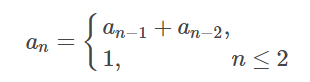又有其通项的计算公式为：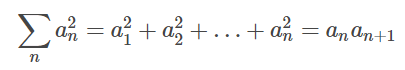则其各项相加的平方和为：

1^2+1^2+2^2+3^2+5^2+8^2=8×13

∑na2n=a21+a22+…+a2n=anan+1
∑nan2=a12+a22+…+an2=anan+1
如果则是，以几何的手段证明：12+12+22+32+52+82=8×1312+12+22+32+52+82=8×13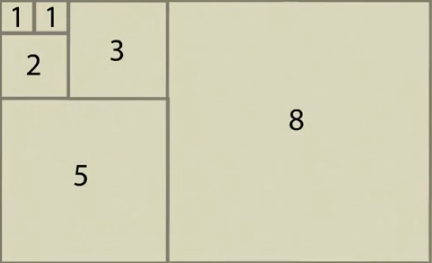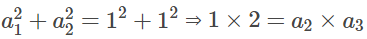2 是 1+1 构成的新的长方形的长；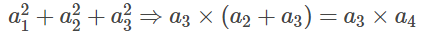a1^2+a2^2得 1*2 的矩形；在此基础上右加上 2^2 的正方形；
展开全文• ## 分部求和法简介

千次阅读 2019-06-27 08:26:23
分部求和法 首先我们知道 ddx(u(x)v(x))=u′(x)v(x)+u(x)v′(x) \cfrac{\mathrm{d}}{\mathrm{d}x}(u(x)v(x))=u&#x27;(x)v(x)+u(x)v&#x27;(x) dxd​(u(x)v(x))=u′(x)v(x)+u(x)v′(x) d(u(x)v(x))=u′(x)v(x)...
• 题目解析3.1 方法一：自下而上、循环求解3.2 青蛙跳台阶、经典递归3.3 fib数列O(logn)O(logn)O(logn)计算3.4 斐波拉契数列通项公式求和公式 1. 题目来源 链接：用两个栈实现队列 来源：LeetCode——《剑指-...递归
• 介绍积分的时候，涉及到了求和，于是我开始推导求和公式。推导后发现初高中时的我简直蠢且懒，不过也挺好玩。初高中数学往往要做序列求和，例如： 那么，这些公式是如何推导出来的呢？如果仅仅是运用初等数学，推导...
• ## C语言:前 n 项求和

千次阅读 2020-02-25 11:29:09
前 n 项求和 算法思路： 1、前n项求和由于第一项和最后一项都已知适合用for循环。 2、当n趋于无穷时，调和数列的极限趋于无穷。 代码如下： #include<stdio.h> int main() { int i; int n; double ...
• 经典的Abel求和法，即Abel变换，是指将有限个两乘积之和，转换成含有其中一部分和的乘积之和的过程。 定理 1 (Abel变换) 设{an}n=0∞\{a_n\}_{n=0}^{\infty}{an​}n=0∞​和{bn}n=0∞\{b_n\}_{n=0}^{\infty}{bn...
• 转载于:https://www.cnblogs.com/linruier/p/9747083.html
• 后来经过推广，我们得到了自然数求和的通式： 我们既然找到了自然数的求和公式，就不妨再去推广一下，试着寻找一下推广的求和公式： 利用二式定理计算 的封闭式为了算这个式子，我们不妨先研究一下这个求和式： ...
• 当需要添加监控为监控交换机总流量的时候，则需要设置监控的...下面是监控的键值，写在上面的公式里 last（）代表最后一次值 avg（，600）代表求十分钟平均值 添加入grafana ，或者也可以制作zabbix 图形 ...
• 高阶等差数列 对于一个给定的数列，将连续两...高阶等差数列都有一个多项式的通项公式。 差分 给定序列aaa，依次求出该序列的kkk阶等差序列，直到某个序列全为000为止，按照下列排列规则排列在纸上 Cn+11 &nb
• 0 序言在求和型数列中，常用的方法是夹逼准则结合定积分知识，这部分内容我会在下一次笔记中更新，本篇主要是介绍一下对于一些特定求和型的数列，我们常见的求和公式以及对这些公式进行不同方法的推导，当作是课外...
• 主要是计算前n和的公式。 前n和的立方公式为 ： s(n)=（n*(n+1)/2）^...公式证明　迭代： 　我们知道： 　0次方和的求和公式ΣN^0=N 即1^0+2^0+...+n^0=n 　1次方和的求和公式ΣN^1=N(N+1)/2 即1^1+...
• 教师：向茂华Page PAGE 3 of NUMPAGES 4 地址：肇嘉浜路91号 电话数列求和的方法1、公式法：如果一个数列是等差、等比数列或者是可以转化为等差、等比数列的数列，我们可以运用等差、等比数列的前n和的公式来求....
• 数列求和1 公式法2错位相减3列相消法4分组求和5倒叙相加1. 求数列,的前和. 2 已知，求的前n和.3. 求数列a,2a2,3a3,4a4,…,nan, …(a为常数)的前n和。4. 求
• 其中 是第 斐波那契数。 有递推公式可知： 则： 因式分解：   泰特展开: 则:  (2) 结合生成函数定义， 公式(1)，可得: 斐波那契数: 2. 特征向量方法： 设存在矩阵M使得 ...斐波那契数
• C++序列求和 ...利用等差数列求和公式比for循坏执行的时间更短 第三个方法就是递归，但递归会保存大量临时数据和重复数据，太多的话会造成栈溢出，程序崩溃 ###方法一：循环累加 #include<iostre.c++
• 幂级数求和函数问题：首先，从牛顿莱布尼茨公式出发，讨论了为什么幂级数要用变限积分，它与不定积分的区别和联系是什么，...最后对于幂级数求和时，起始n和角标的规定进行讨论，给出了幂级数和函数的思路及步骤。
• 积分推导正整数平方和公式积分推导正整数平方和公式思路证明过程 积分推导正整数平方和公式 正整数平方和公式： 12+22+32+⋯+n2=n(n+1)(2n+1)6 \Large 1^2 + 2^2 + 3^2 + \cdots + n^2 = \frac{n(n+1)(2n+1)}{...
• 后来百度了之后,原来可以通过立方差公式简单求解,不过还是发表记录一下,当时自己的一顿瞎推导
• 多项式求和 Problem Description 多项式的描述如下： 1 - 1/2 + 1/3 - 1/4 + 1/5 - 1/6 + ... 现在请你求出该多项式的前n的和。   Input 输入数据由2行组成，首先是一个正整数m（m   Output 对于...
• ## 裂项法

千次阅读 2013-04-07 20:02:53
项法的实质是将数列中的每（通）分解，然后重新组合，使之能消去一些，最终达到求和的目的. 通分解（裂） （1）1/[n(n+1)]=1/n-1/(n+1) （2）1/[(2n-1)(2n+1)]=1/2[1/(2n-1)-1/(2n+1)] （3）1/[n...数学知识 排列组合
• 总是看到有人问递推的通项公式如何求，母函数（Generating function）是一个很好用的工具， 现总结如下以供学习  母函数是组合数学里面的概念，其实就是这坨东西  是不是看不明白，确实有些生疏，再看这一坨 ...function 工具 c
• 提示：若第一项为a , 以后每一由前一乘以10加上a递推得到，然后求和。 其实这题就是对递归思想的很简单的一个应用，代码也不长，也不难，大家就自己看源码吧。 源码： #include<iostream> using ...递归算法...